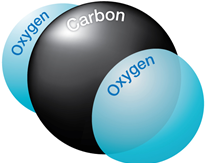# Effect of CO2 on downhole flowrate calculation

Downhole flow rate can be calculated from surface flow rate (stock tank barrels) using the following equation. It is assumed that no gas is dissolved in the water phase and the water formation volume factor is equal to one.

Downhole flow rate = [(Oil rate)sc × Bo] + [(Free GOR) × (Oil rate)sc × Gas FVF] + (Water rate)sc

Free GOR = Producing GOR – Solution GOR, therefore:

## q = ( Qo × Bo ) + [ ( R – Rs) × Qo × Bg × 1000] + Qw

Where:

• q = downhole flow rate (bbl/d or m3/d)
• Qo = Oil flow rate at standard conditions (stb/d or m3sc/d)
• Bo = Oil formation volume factor (bbl/stb or m3sc/m3sc)
• R = Producing gas-oil ratio (scf/stb or m3sc/m3sc)
• Rs = Solution gas-oil ration (scf/stb or m3sc/m3sc)
• Bg = Gas formation vol. factor (bbl/mscf or m3sc/m3sc)
• Qw = Water flow rate at standard conditions (stb/d or m3sc/d)

### Effect of CO2 on downhole flowrate calculation:If CO2 is present, the calculation of downhole flow rate becomes more complex for many reasons:

Firstly, CO2 has its own Bg which is different from that of hydrocarbon gas. Also, while hydrocarbon gas does not dissolve into water, CO2 does. Thus, the Bw term becomes significant. Furthermore, oil formation volume factor (Bo) changes when CO2 is dissolved into it (because CO2 causes oil swelling). In addition, CO2 gas is generally dissolved into the water before it dissolves into the oil. Also, the oil must accept all available hydrocarbon gas before any CO2 can be put into oil solution.

Along 18 step procedure is used to calculate downhole flow rate when CO2 is present. The final equation looks like the following equation:

## q = (Qo × Bo × OSF) + (WCO × Bw) + WU + (FHC × Bg) + (FCO × B’g)

Where:

• q = downhole flow rate (bbl/d or m /d)
• Qo = Oil flow rate at standard conditions (stb/d or m3sc/d)
• Bo = Oil formation volume factor (bbl/stb)
• OSF = Oil Swell Factor
• WCO = Water flow rate affected by CO2 (stb/d or m3sc/d)
• WU = Water flow rate unaffected by CO2 (stb/d or m3sc/d)
• FHC = Free gas flow rate (mscf/d or m3sc/d)
• Bg = Gas formation volume factor (bbl/mscf or m3/m3sc)
• FCO = Free CO2 gas flow rate (mscf/d or m3sc/d)
• Bg’ = CO2 gas formation volume factor (bbl/mscf or m3/m3sc)

When CO2 is present, it becomes critically important to convert from stock tank barrels to reservoir barrels prior to designing any artificial lift systems. Errors are as high as two to one can result if this is not done. Fortunately, most modern design software do this conversion prior to sizing submersible pumps.